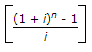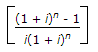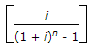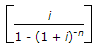# Civil Engineering - Engineering Economy

### Exercise :: Engineering Economy - Section 2

6.

The owner of the construction company makes use of the estimate :

 A. to determine the capital investment costs. B. to assist in financial arrangements C. to determine economic feasibility of the project. D. to determine the tax, insurance and evaluation purpose. E. All of these

Explanation:

No answer description available for this question. Let us discuss.

7.

Which one of the following is not a construction estimate ?

 A. Initial feasibility estimate B. Conceptual preliminary budget C. Definite estimate D. None of these

Explanation:

No answer description available for this question. Let us discuss.

8.

Pick up the correct statement from the following:

 A. Uniform series compound amount factor =B. Uniform series present worth factor =C. Sinking fund factor =D. Capital recovery factor =where letters carry their usual meanings. E. All of these

Explanation:

No answer description available for this question. Let us discuss.

9.

Pick up the method used for project evaluation and selection in capital budgetting from the following:

 A. pay back period B. Internal ratio of return C. Net present worth D. Profitability index E. All the above

Explanation:

No answer description available for this question. Let us discuss.

10.

Pick up the correct statement from the following:

 A. The ratio of current assests, loans and advances, and the current liquidity is called current ratio. B. Larger the current ratio, larger is the margin of safety. C. The operating profit is the difference between gross profit and operating expenses. D. All of these